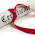# A number that is 5 times the sum of its digits

Question: There is a number that is 5 times the sum of its digits. What is this number? Answer is not0.

The number is 45, simply because
45 = 5 * (4 + 5)
How does one find this number?
Let T be the digit in the tens place and U be the digit in the units place. Then, the
number is 10*T + U, and the sum of its digits is T + U.
The following equation can be readily written:
10*T + U = 5*(T + U) or
10*T + U = 5*T + 5*U or
5*T = 4*U
Thus, T / U = 4 / 5
Since T and U are digits, T must be 4 and U must be 5.